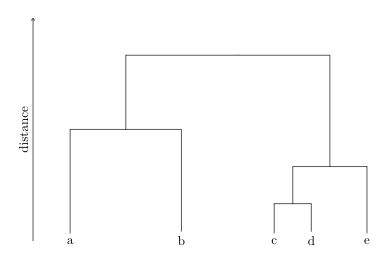Instantly share code, notes, and snippets.

# dahoo/dendrogram.tex

Last active August 28, 2022 23:14
Hierarchical Clustering Dendrogram in Latex
This file contains bidirectional Unicode text that may be interpreted or compiled differently than what appears below. To review, open the file in an editor that reveals hidden Unicode characters. Learn more about bidirectional Unicode characters
 \begin{tikzpicture}[sloped] \node (a) at (-6,0) {a}; \node (b) at (-3,0) {b}; \node (c) at (-0.5,0) {c}; \node (d) at (0.5,0) {d}; \node (e) at (2,0) {e}; \node (ab) at (-4.5,3) {}; \node (cd) at (0,1) {}; \node (cde) at (1,2) {}; \node (all) at (-1.5,5) {}; \draw (a) |- (ab.center); \draw (b) |- (ab.center); \draw (c) |- (cd.center); \draw (d) |- (cd.center); \draw (e) |- (cde.center); \draw (cd.center) |- (cde.center); \draw (ab.center) |- (all.center); \draw (cde.center) |- (all.center); \draw[->,-triangle 60] (-7,0) -- node[above]{distance} (-7,6); \end{tikzpicture}

### jendrikw commented May 29, 2019

If you use \coordinate instead of \node, you don't need the .center.

### sunandabansal commented Jul 17, 2021

Thank you for sharing this gist. I was getting an error because of -triangle 60 in line 21, so I removed that part -

\begin{tikzpicture}[sloped]
\node (a) at (-6,0) {a};
\node (b) at (-3,0) {b};
\node (c) at (-0.5,0) {c};
\node (d) at (0.5,0) {d};
\node (e) at (2,0) {e};
\node (ab) at (-4.5,3) {};
\node (cd) at (0,1) {};
\node (cde) at (1,2) {};
\node (all) at (-1.5,5) {};

\draw  (a) |- (ab.center);
\draw  (b) |- (ab.center);
\draw  (c) |- (cd.center);
\draw  (d) |- (cd.center);
\draw  (e) |- (cde.center);
\draw  (cd.center) |- (cde.center);
\draw  (ab.center) |- (all.center);
\draw  (cde.center) |- (all.center);

\draw[->] (-7,0) -- node[above]{distance} (-7,6);
\end{tikzpicture}

And the result was (for reference) -### sunandabansal commented Jul 18, 2021

A little enhance version of the above script -

\begin{tikzpicture}[sloped]
\node (a) at (-6,0) {a};
\node (b) at (-3,0) {b};
\node (c) at (-0.5,0) {c};
\node (d) at (0.5,0) {d};
\node (e) at (2,0) {e};
\node (ab) at (-4.5,3) {};
\node (cd) at (0,1) {};
\node (cde) at (1,2) {};
\node (all) at (-1.5,4.5) {};

\draw  (a) |- (ab.center);
\draw  (b) |- (ab.center);
\draw  (c) |- (cd.center);
\draw  (d) |- (cd.center);
\draw  (e) |- (cde.center);
\draw  (cd.center) |- (cde.center);
\draw  (ab.center) |- (all.center);
\draw  (cde.center) |- (all.center);

\draw[<-] (-10,0) -- node[above]{similarity} (-10,5);
\draw[->] (-9,0) -- node[above]{distance} (-9,5);

\draw (-7,0) -- (-7,5);

\foreach \y in {0,1,2,3,4,5}
\draw[shift={(0,\y)},color=black] (-7,0) -- (-7.1,0);

\node[left] at (-7.1,0) {$1.0$} ;
\node[left] at (-7.1,1) {$0.8$} ;
\node[left] at (-7.1,2) {$0.6$} ;
\node[left] at (-7.1,3) {$0.4$} ;
\node[left] at (-7.1,4) {$0.2$} ;
\node[left] at (-7.1,5) {$0.0$} ;

\draw[dashed,color=gray] (-7,3.5) -- node[at end, right]{cut-off} (2.5,3.5);
\draw[color=gray] (-7,3.5) -- (-7.1,3.5) node[left] {$0.3$} ;
\end{tikzpicture}